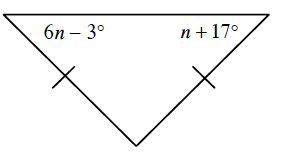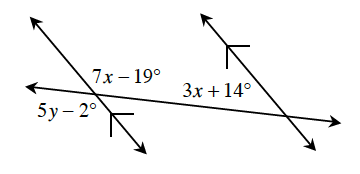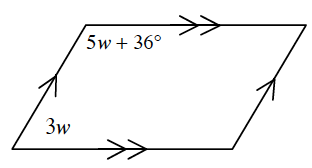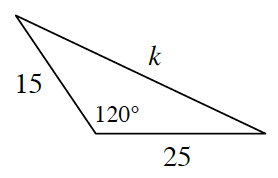### Home > CCG > Chapter 7 > Lesson 7.1.3 > Problem7-37

7-37.

Examine the relationships in the diagrams below. For each one, write an equation and solve for the given variable(s). Show all work.

1.Since the triangle is isosceles, the angles shown are equal.

$n=4°$

1.First solve for $x$, then $y$. Refer to the Math Notes box in Lesson 2.1.4 if you need help remembering any specific angle pair relationships.

1.Same side interior angles are supplementary.

$w=18°$

1.Use the Law of Cosines to solve for $k$.